Courses

# Conservation of Energy and Momentum (Part - 1) Electrical Engineering (EE) Notes | EduRev

## Electromagnetic Theory

Created by: Machine Experts

## Electrical Engineering (EE) : Conservation of Energy and Momentum (Part - 1) Electrical Engineering (EE) Notes | EduRev

The document Conservation of Energy and Momentum (Part - 1) Electrical Engineering (EE) Notes | EduRev is a part of the Electrical Engineering (EE) Course Electromagnetic Theory.
All you need of Electrical Engineering (EE) at this link: Electrical Engineering (EE)

Coulomb Gauge and the Potential formulation of Maxwell’s equations

The Maxwell’s equations in their final form are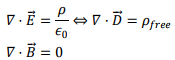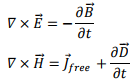We had, in the last lecture, made a reformulation of these equations in terms of scalar and vector potentials. This gave us two “coupled” equations for four quantities, i.e. 3 components of the vector potential and one component of scalar potential. We had seen that these equations get decoupled in Lorentz gauge.

We had a lot of discussion on Coulomb gauge in which the divergence of the vector potential is zero. Is this gauge any good to be used now?

Recall the pair of equations,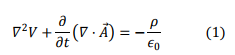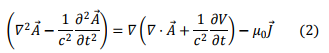Note that first equation gets decoupled in Coulomb gauge and becomes a Poisson’s equation for the scalar potential with the formal solution,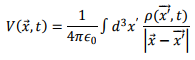The second equation is not straightforward and requires a lot of mathematical mannipulation before the decoupling can be seen.

To avoid repeating the same expression unnecessarily, we will concentrate on the right hand side of eqn. (2). In Coulomb gauge the term with the divergence drops out and the right hand side of (2) becomes,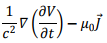We insert the formal solution of the scalar potential into this equation,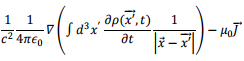Now the term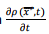is a current and we replace it by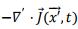using the equation of continuity and the term becomes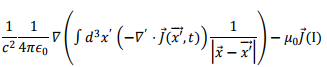We will do some further simplification to (I). But first let us recall that we have learnt that a vector is completely determined when its divergence and curl are specified. This allows us to write the current density vector inside the integral as a sum of one part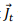whose divergence is zero and another part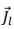whose curl is zero,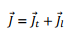Using the identity,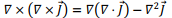we can write,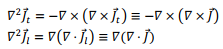where we have used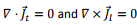in arriving at both these relations. We will shortly return to using these relations.

Let us get back to the relation (I). Note that the gradient outside is taken with respect to the point of observation. We can take it inside the integration and it will act only on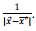However, since this depends only on the difference of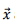and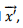we can replace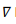by'by incorporating a minus sign. With this (I) becomes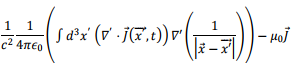We will simplify this further by using chain rule differentiation,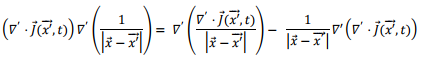Substituting this into the preceding term, we have two integrals, one of which is a volume integral of a gradient. This term can be converted to a surface integral like the way we do for the divergence theorem and take to surface to infinity to make this term zero. This implies the remaining integral is over all space and we get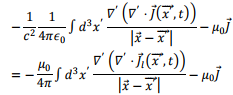in obtaining this step, we have used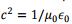and used the fact that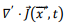can be written as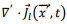because the transverse part has zero divergence. Further, we can use the fact that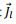is irrotational to write,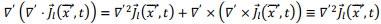With this (I) takes the form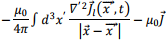At this stage, we will use the Green’s identity for fields T and U according to which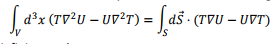As our fields vanish at infinity, we have,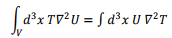Using this, we get,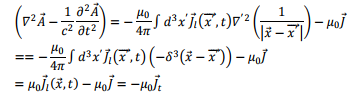Thus the original equation for the vector potential has now been completely decoupled from the scalar part and we have , instead, an inhomogeneous wave equation,Electromagnetic Momentum

We have seen that the electromagnetic field carries energy. A natural question arises as to whether it carries momentum as well. The answer is affirmative and we will illustrate this by a simple procedure. A more rigorous derivation requires use of the theory of relativity. Suppose, we have two charged particles, q1 and q2, the former moving along the x axis while the latter moves along the y axis.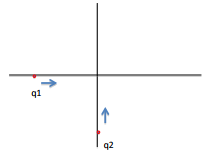The force on q1 just as it passes by the origin is purely electrical as it lies along the direction of motion of q2, and is given by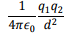where d is the distance between the charges at that instant. This is also the magnitude of the electric force on q2 due to q1. However, if we look at the force on q2 due to q1, there is also a magnetic force. This is because the moving charge q1 creates a magnetic field in the z direction which exerts a force on q2. This is certainly anomalous and is in apparent violation of the third law. We say it is an apparent violation because the third law is essentially a statement of conservation of momentum and it is the total momentum of the system that needs to be conserved. In this case, in addition to the two charges, there exists the electromagnetic field and if the field itself carries momentum, there is no violation. This is intuitive but the fact that electromagnetic field carries momentum is a fact.

Let us look at the force exerted on a system of sources (charges and currents) and electromagnetic field. Let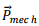represent the moment associated with the sources. The force exerted on the system of sources is then given by Lorentz force equation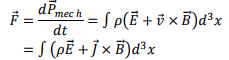Once again, for simplicity, we will consider linear electric and linear magnetic material. We will use Maxwell’s equations to cast these equations in in terms of field variables. We replace ρ sing Gauss’s law and the current density from Ampere’s law,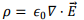and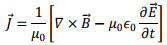Substituting these in the force equation, we get,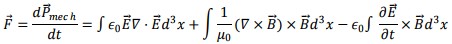Let us simplify some of the terms,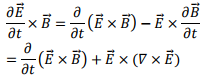In the last expression we have used Faraday’s law. Thus we have,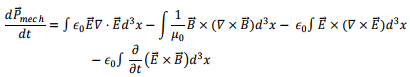In the second term we have changed the order of cross product and hence a minus sign.

The last term on the right can be seen to be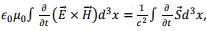where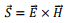is the Poynting vector. Since the expression has the dimension of force,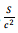has the dimension of momentum density and we will identify this term as the rate of change of momentum associated with the electromagnetic field (radiation field) and take it to the left hand side to be added to the rate of change of momentum of the sources Prad.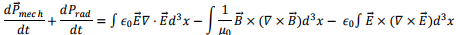The expression on the right looks asymmetric in the electric and magnetic quantities which can be rectified easily. Let us look at the electric field terms represented by the first and the third terms on the right.

The integrand is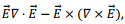other than for a factor of ∈In order to simplify this to desired form, we will calculate the Cartesian components of this. Let us find what its x component is and then we will add up the three components.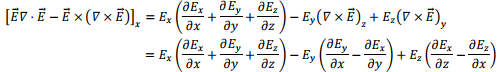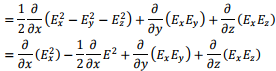From symmetry, one can write the y and z components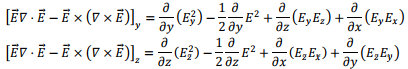Let us add the three components. The second term of each of the expressions add to give us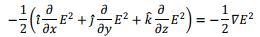The remaining terms look messy and we will return to them shortly.

Let us consider the magnetic field terms. In order to make it symmetrical with the magnetic field, we need to add a term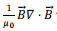to the triple vector product term. This is simply adding zero because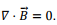However, this will make the electric and the magnetic fields at par and we would get a contribution of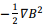similar to the case of the electric field. This would give us a term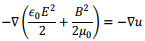where u is the energy density of electromagnetic field. Thus this term (i.e. the gradient of the energy density) represents the momentum density term.

We will return to a discussion of the remaining terms in the next lecture.

Offer running on EduRev: Apply code STAYHOME200 to get INR 200 off on our premium plan EduRev Infinity!

,

,

,

,

,

,

,

,

,

,

,

,

,

,

,

,

,

,

,

,

,

;